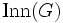# Cyclic automorphism group implies abelian

This article gives a result about how information about the structure of the automorphism group of a group (abstractly, or in action) can control the structure of the group
View other such results

## Statement

Suppose$G$ is a group that is an Aut-cyclic group (?): the Automorphism group (?)$\operatorname{Aut}(G)$ is a Cyclic group (?). Then,$G$ is an Abelian group (?).

## Proof

Given: A group$G$. The automorphism group$\operatorname{Aut}(G)$ is cyclic.

To prove:$G$ is abelian.

Proof:

Step no. Assertion/construction Facts used Given data used Previous steps used Explanation
1 Let$Z$ be the center of$G$. Then,$G/Z$ is isomorphic to a subgroup of$\operatorname{Aut}(G)$, called the inner automorphism group$\operatorname{Inn}(G)$. Fact (1) [SHOW MORE]
2$G/Z$ is a cyclic group. Fact (2)$\operatorname{Aut}(G)$ is cyclic Step (1) Step-fact-given combination direct.
3$G$ is abelian Fact (3) Steps (1), (2) Fact-step combination direct.